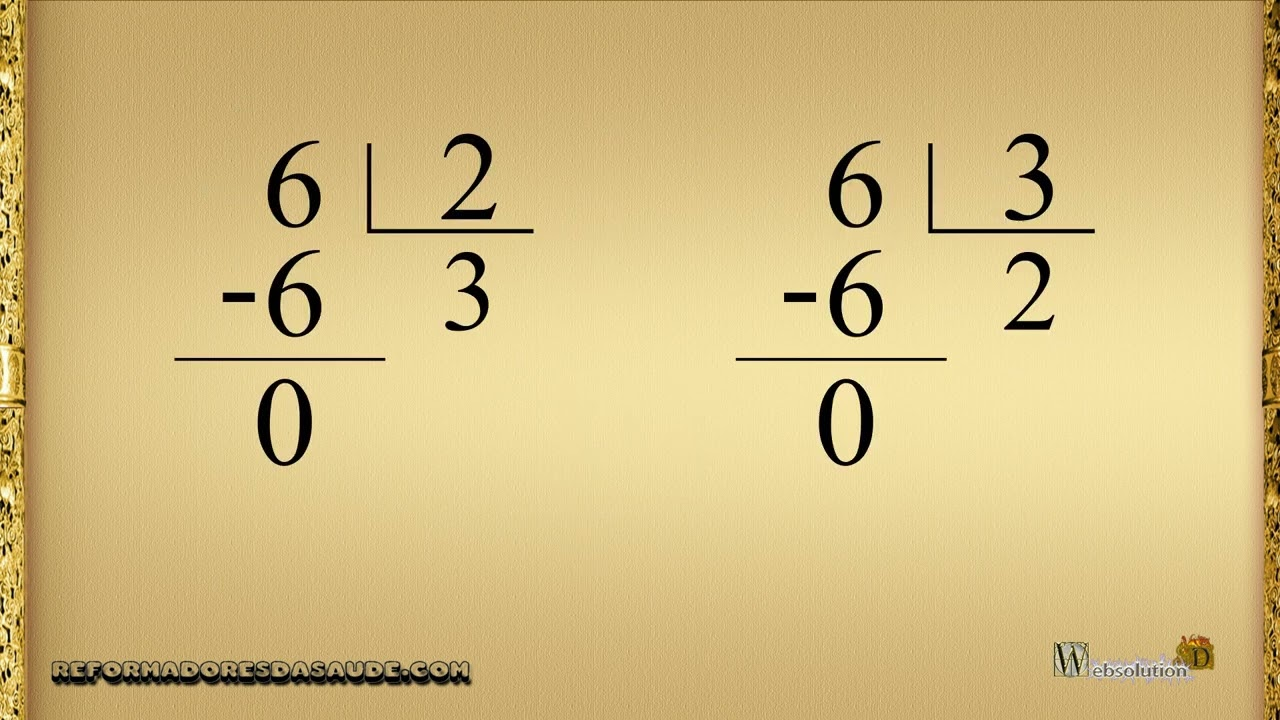# Prime numbers

Código FM06-E4850-I

##### VIEW:306 DATA:0000-00-00
What are prime numbers? Prime numbers are numbers that can only be divided by themselves, so there is no remainder.
For example, 3 is a prime number, but if you divide it by 2, it will give you 1 as a remainder. But if you divide by 3, there is no remainder.
The number 4 is not prime, because it is divided by 2 so there is no remainder. Remember that a prime number is a number that is only divided by itself and that has no remainder.
And the number 5, 5 divided by 2 gives 1 remainder. If dividing by 3 gives 2 remainder, if dividing by 4 gives 1 remainder, but if dividing by 5, in this case there is no remainder.
The number 6 is not prime, because if divided by 2, there is no remainder, and if divided by 3, there is no remainder.
But the number 7 is prime, because divided by 2 gives remainder 1, divided by 3 gives remainder 1, divided by 4 gives remainder 3, divided by 5 gives remainder 2, divided by 6 gives remainder 1, but if divide by 7 gives no remainder.
So we see that the prime number is a number that, in order to have no remainder, can only be divided by itself.
Some interesting features, the number 1, is called the neutral element of multiplication, so it divides all numbers, and even then prime numbers are still called prime.
Another interesting thing is that you can't find prime numbers without doing tests to see if it's prime. Look at the work that went into figuring out that the number 7 is prime. Imagine a large prime number, like 7919.
So-called cryptocurrencies, and encrypted conversations are encoded by huge prime numbers, discovered through supercomputers.
Thus, codes have prime numbers as their base. Many companies seek the largest prime numbers to achieve the greatest security in their communications, and encryptions of sensitive data.
In this way, prime numbers are not only special numbers but also extremely useful in our modern world.Visite o nosso canal youtube.com/buscadaverdade e se INSCREVA agora mesmo! Lá temos uma diversidade de temas interessantes sobre: Saúde, Receitas Saudáveis, Benefícios dos Alimentos, Benefícios das Vitaminas e Sais Minerais... Dê uma olhadinha, você vai gostar! E não se esqueça, dê o seu like e se INSCREVA! Clique abaixo e vá direto ao canal!

#### Saiba Mais

•Nutrição
Vegetarianismo e a Vitamina B12
•Receita
Como preparar a Proteína Vegetal Texturizada
•Arqueologia
Livro de Enoque é um livro profético?
•Profecia
O que ocorrerá no Armagedom?

#### Tags

prime numbers, passwords, cryptocurrencies, encoding, security, dividers